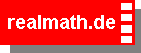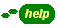In this math program you will practice dividing fractions. Therefore a random generator created a problem.

Your task is to solve and maybe simplify it.
Look at the Example.

Example:To validate your answer,
click on the button  validate .

To create a new problem,
click on the button  create problem .

Can you top
279 points ?## All about fractions - dividing fractions### random problem - level 3-

 ÷ = × = =

Score:  Problems:

© Andreas Meier, Ph.D., Sophie-Scholl-Realschule Weiden i.d.OPf